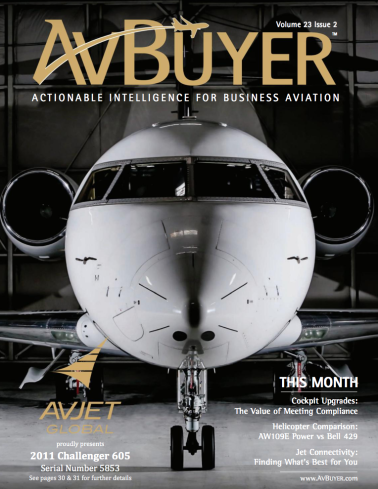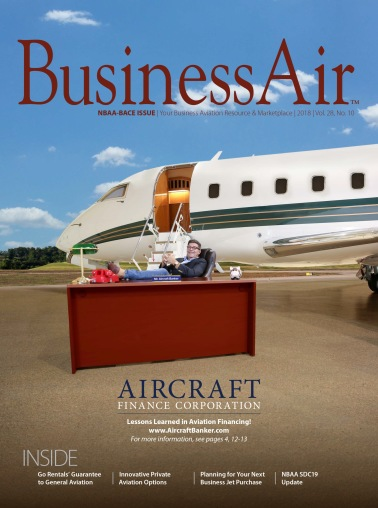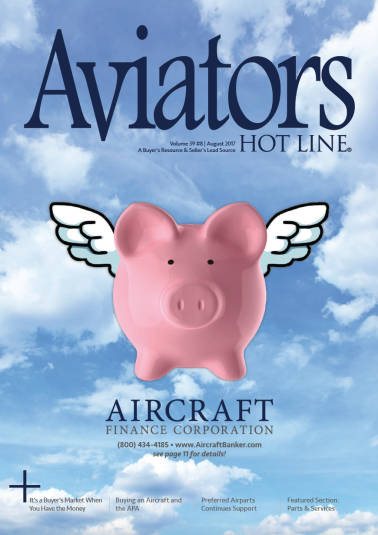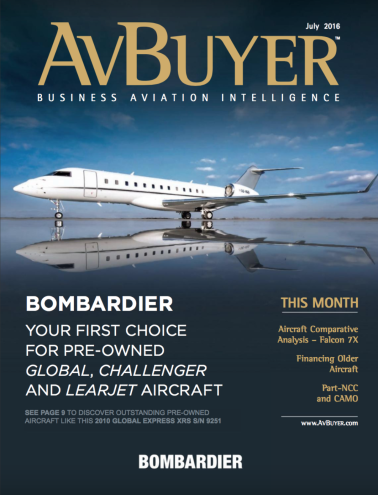# Recent Transactions

AFC is long on transactions, but our focus is on the relationship. There’s a reason why we have lifelong clients.#### 2016 Pilatus PC-12

With an interest rate of 3.43%, this transaction was structured with a 240-month term with a 90% Advance Rate.

#### 2013 Gulfstream G280

With an interest rate of 3.63%, this transaction was structured with a 240-month term with a 80% Advance Rate.

#### 2011 Phenom 100

With an interest rate of 4.11%, this transaction was structured as a 84-month term with a 240-month amortization.

#### 2020 Pilatus PC-12NG

With an interest rate of 4.66%, this transaction was structured as a 120-month term with a 240-month amortization.

#### 2001 Hawker 800XP

With an interest rate of 3.99%, this transaction was structured with a 180-month term with a 80% Advance Rate.

#### 2003 Falcon 900EX

With an interest rate of 4.4%, this transaction was structured as a 84-month term with a 240-month amortization.

#### 2014 Challenger 350

With an interest rate of 3.43%, this transaction was structured as a 60-month term with a 240-month amortization.

#### 2008 Hawker 850XP

This transaction was structured as a 60-month term with a 240-month amortization, at an interest rate of 5.11%.

#### 1996 Pilatus PC12

With an interest rate of 4.99%, this transaction was structured as a 120-month term with a 240-month amortization.

#### 2001 Citation Encore 560

With an interest rate of 4.88%, this transaction was structured as a 120-month term with a 240-month amortization.

#### 1991 Citation VI

With an interest rate of 4.88%, this transaction was structured as a 120-month term with a 240-month amortization.

#### 1999 Hawker 800XP

This transaction was structured as a 120-month term with a 240-month amortization, at an interest rate of 5.24%.

#### 2007 Hawker 400A

With an interest rate of 4.88%, this transaction was structured as a 120-month term with a 240-month amortization.

#### 2011 G200

This transaction was structured as a 120-month term with a 240-month amortization, at an interest rate of 5.11%.

#### 2009 Hawker 900XP

This transaction was structured as a 120-month term with a 240-month amortization, at an interest rate of 5.11%.

#### 2014 King Air 350

This transaction was structured as a 60-month term with a 180-month amortization, at an interest rate of 4.75%.

#### 2009 Challenger 605

This transaction was structured as a 120-month term with a 240-month amortization, at an interest rate of 4.49%.

#### 2008 Hawker 900XP

With an interest rate of 4.75%, this transaction was structured as a 60-month term with a 240-month amortization.

#### 2002 Citation Excel

With an interest rate of 5.25%, this international transaction was structured as a 60-month term.## We Are Proud Members Of:## Recent NewsRead our new article on page 46 in the February issue of AvBuyer.Ever wondered who Mr. Arcraft Banker is? Click and find out!